Next: Projective Bases Up: Projective Mappings Previous: Example:

#### Camera calibration:

Assuming that the camera performs a exact perspective projection (see 1.1.2), we have seen that the image formation process can be expressed as a projective mapping from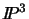to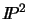. Projective camera calibration is the computation of the projection matrix associated with this mapping. This is usually done using a set of points whose 3D locations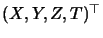are known. If a pint projects to pixel coordinates (u,v), the projection equations can be written: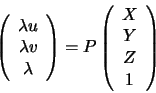Taking ratios to eliminate the unknown scale factor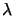, we have:

 u =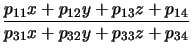v =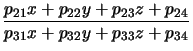(2.1)

As P is only defined up to an overall scale factor, this system has 11 unknowns. At least 6 points are required for a unique solution, but usually many more points are used in a least squares optimization that minimizes the effects of measurement uncertainty.

The projection matrix P contains both interior and exterior camera parameters. We will not consider the decomposition process here, as the exterior orientation/interior calibration distinction is only meaningful when projective space is reduced to Euclidean.

Exercise 2.2   : Assuming perspective projection centered at the origin onto plane z=1, and a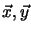image reference frame corresponding to thedirections of the 3D reference frame, prove that the projection matrix P has the form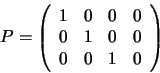The null space of P (the set of X such that PX=0) corresponds to which 3D point X? What does the 3D point (x, y, 0) project to?Next: Projective Bases Up: Projective Mappings Previous: Example:
Bill Triggs
1998-11-13